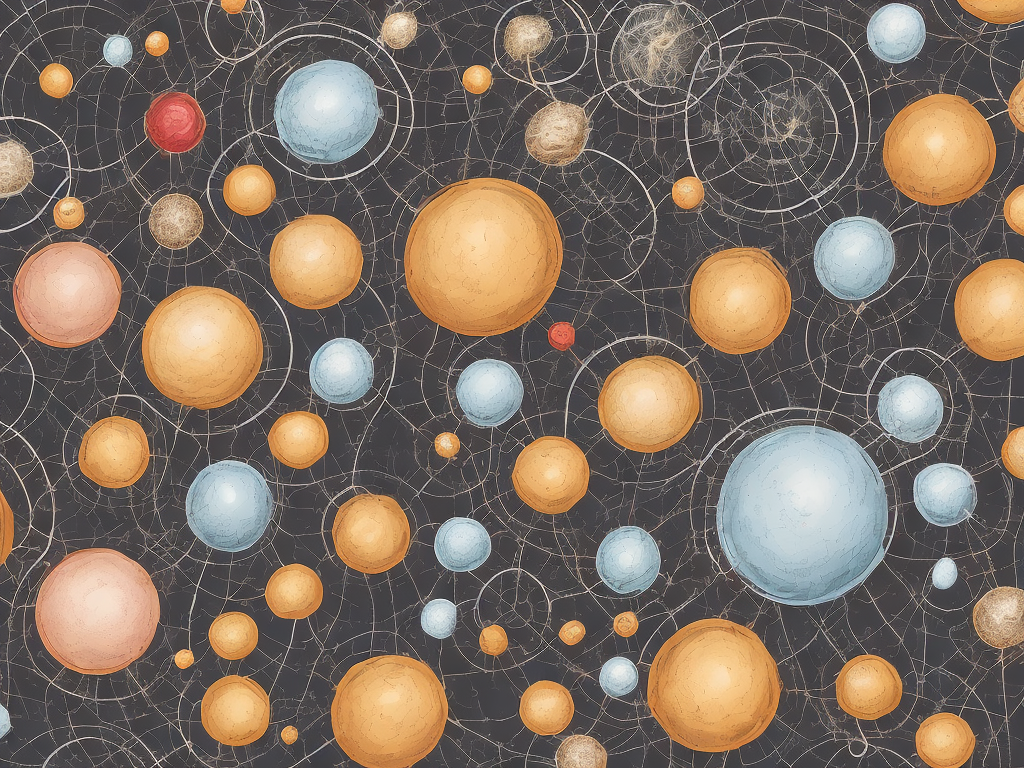# Difference Between Independent And Dependent Variables

tl;dr
The independent variable is the factor that is manipulated or controlled by the researcher, while the dependent variable is the outcome or result of manipulating the independent variable.The Difference Between Independent And Dependent Variables

When conducting research, it is essential to understand the difference between independent and dependent variables as they play a crucial role in the design and interpretation of experiments. Independent and dependent variables are two key concepts in the scientific method that help researchers examine relationships, establish cause and effect, and study the effects of various factors on a phenomenon. In this article, we will delve into the definitions and distinctions between these two variables, and explore their significance in scientific research.

To begin with, let us define independent and dependent variables. An independent variable is a variable that researchers deliberately manipulate or control in an experiment. It is the factor that researchers impose on the subjects or conditions under study to observe the effect it has on the dependent variable. The independent variable is also known as the manipulated variable. It is controlled and set by the researcher and remains unchanged throughout the course of the experiment.

On the other hand, a dependent variable is the variable that researchers measure or observe as the outcome or result of manipulating the independent variable. It is the variable that researchers are interested in understanding or explaining. Unlike the independent variable, the dependent variable is not under the direct control of the researcher. It is influenced by changes in the independent variable and can vary based on its values.

To better comprehend the relationship between independent and dependent variables, let us consider an example. Suppose a scientist is interested in studying the effect of caffeine on the heart rate of individuals. In this scenario, the independent variable would be the amount of caffeine consumed by the participants. The researcher can manipulate the levels of caffeine intake by dividing subjects into different groups, each receiving a specific dose of caffeine. The dependent variable, in this case, would be the heart rate of the individuals. The scientist would measure the heart rate of each subject before and after the caffeine consumption to observe any changes or patterns that may occur.

It is crucial to note that the cause and effect relationship between independent and dependent variables is fundamental in experimental research. The independent variable is considered the cause or the factor that leads to changes in the dependent variable, which is the effect. By manipulating the independent variable, researchers aim to determine whether it has a significant impact on the dependent variable. This cause and effect relationship allows researchers to establish causal relationships and make inferences about the phenomena under investigation.

In addition to understanding the definitions and relationship between independent and dependent variables, it is important to recognize how these variables are distinguished in terms of their characteristics and roles within an experiment.

Firstly, independent variables are distinguished by their values and attributes. They can be divided into two main types: categorical and continuous variables. Categorical variables are those that have distinct categories or groups. For example, in a study examining the effect of education level on job satisfaction, the independent variable would be education level, which can be categorized into groups such as high school, college, or postgraduate education. On the other hand, continuous variables are those that have a range of values. For instance, in a study investigating the effect of temperature on plant growth, the independent variable would be the temperature, which can vary from low to high values.

Dependent variables, on the other hand, are typically continuous variables. They represent the outcome or result of the manipulation of the independent variable. In most cases, dependent variables are measured using instruments or tools that provide numerical data. Researchers often collect data on dependent variables before and after the manipulation of the independent variable to analyze changes and draw conclusions about the relationship between the two.

Secondly, the roles of independent and dependent variables in an experiment differ. As previously mentioned, researchers manipulate the independent variable to observe its effect on the dependent variable. The independent variable is the factor that researchers intentionally change or control to examine whether it has an impact on the dependent variable. By manipulating the independent variable, researchers can vary conditions and compare different groups to assess the effects of the independent variable on the dependent variable.

On the other hand, the dependent variable is the one that researchers measure, observe, or record as the outcome of manipulating the independent variable. It is the variable that researchers want to explain or understand based on the changes in the independent variable. The dependent variable provides valuable data and insights that help researchers draw conclusions and make inferences about the effect of the independent variable.

It is important to ensure that independent and dependent variables are clearly defined and operationalized in research studies to maintain clarity and consistency. It is also crucial to consider potential confounding variables that might affect the relationship between the independent and dependent variables. Confounding variables are those that are not controlled or measured but can influence the results of the experiment. Identifying and controlling for confounding variables is essential to establish a valid cause and effect relationship between the independent and dependent variables.

In conclusion, independent and dependent variables are fundamental concepts in scientific research. While the independent variable is manipulated or controlled by the researcher, the dependent variable is measured or observed as the outcome of the manipulation. These variables play a vital role in establishing cause and effect relationships, studying the effects of certain factors, and understanding the relationships between different phenomena. Understanding the distinction between independent and dependent variables is essential for researchers to design experiments, collect data, and draw meaningful conclusions about the variables under investigation.